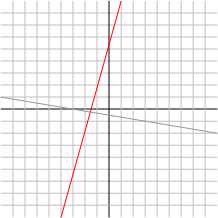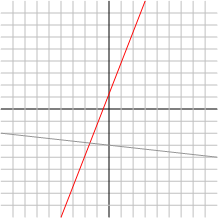Classrooms Curricula References Textbooks Site Map

# The Slope and Y-intercept

## The SlopeThe slope of a line is a measure of how steep the line is. A line that climbs quickly, like the red line in the picture to the right, has a big slope, while a line that climbs slowly has a small slope.

By "small", I mean "close to zero" not "very negative", e.g. -1,000,000. A negative slope means something else. A line whose slope is negative is "decreasing". It starts from the top left corner and drops to the top right. A positive slope, on the other hand, means the line is "increasing", i.e. it starts at the bottom left and rises to the top right.

Putting these two ideas together gives us the following possibilities. (Remember that "small" means close to zero.)

 size sign behavior small positive the line is relatively flat and increasing small negative the line is relatively flat and decreasing big positive the line is relatively steep and increasing big negative the line is relatively steep and decreasing

### Calculating the Slope

The last section's descriptive definitions were interesting but it would be great if we could turn the slope into a number instead of subjective words like "big" and "flat". The idea behind the slope's equation is a simple one

 m = rise run

"rise" is pretty self-explanatory. It's how much the line increases between two points. In other words, how much it changes in the y direction. "run", on the other hand, refers to how far the line moves in the x direction between the same two points.

That's nice but we still haven't translated our definition into a number. To do that we have to have real points with which to work. Say I give you two points whose coordinates are (x1, y1) and (x2, y2). Then the line changes by y1 - y2 in the y direction and x1 - x2 in the x direction. Substituting these expressions into our "rise over run" formula gives us

 m = y1 - y2 x1 - x2

For some examples of calculating the slope with real numbers see the Getting the Equation section.

## The Y-interceptThis is the easier of the two parts of a line's equation. The y-intercept is just the point where the line crosses the y-axis. If you look at the graph on the right, the y-intercept of the red line is 1 and the y-intercept of the gray line is -3. Notice that the y-intercept is always given as a number not a set of coordinates.

Does it look to you like the red line crosses the y-axis at 1? Maybe it's really 1.1 and the graph is just hard to read because of the steepness of the line. This is always a problem when dealing with visual graphs. You're always better off using the equation instead of a picture unless a question specifically tells you otherwise. If a question specifies that you should use a diagram then you can assume that the person asking it is going to take minor inaccuracies into account.